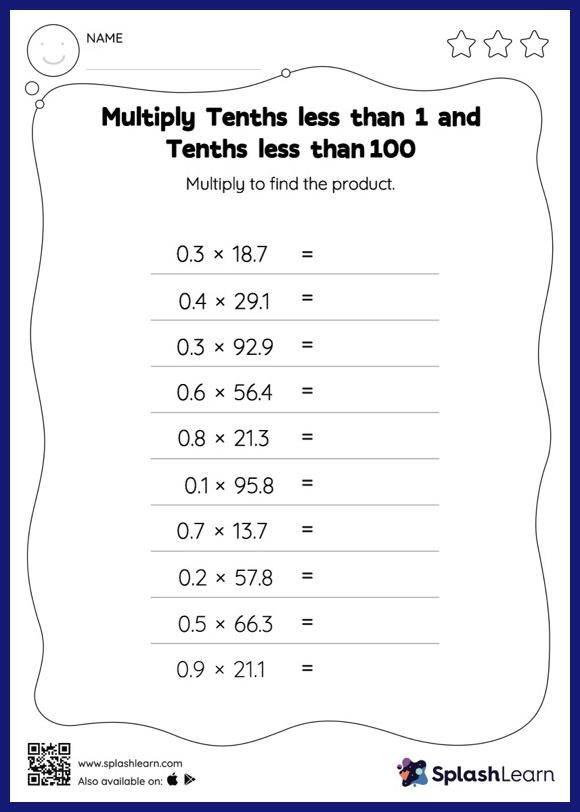# Multiply Tenths less than 1 and Tenths less than 100: Horizontal Multiplication Worksheet

Home > Multiply Tenths less than 1 and Tenths less than 100: Horizontal MultiplicationA tenth multiplied by another tenth will result in a product with two decimal digits. As they practice this idea in multiply tenths less than 1 and tenths less than 100 worksheet, they first multiply the numbers without accounting for the decimal points and then add the decimal point two places from the right in the product. As the worksheet uses a horizontal format, it allows for more creativity when it comes to how the student solves the problem. In contrast, in the vertical format, students usually employ the standard method to solve.